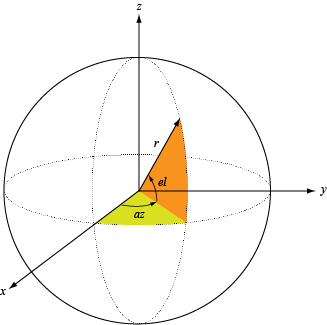# directivity

Compute array directivity

## Syntax

``D = directivity(array,FREQ,ANGLE)``
``directivity(___,Name,Value)``

## Description

````D = directivity(array,FREQ,ANGLE)` returns the Directivity (dBi) of an array of antenna or microphone elements, `array`, at frequencies specified by `FREQ` and in angles of direction specified by `ANGLE`.The integration used when computing array directivity has a minimum sampling grid of 0.1 degrees. If an array pattern has a beamwidth smaller than this, the directivity value will be inaccurate.```
````directivity(___,Name,Value)` plots the array pattern with additional options specified by one or more `Name,Value` pair arguments.```

## Input Arguments

collapse all

Phased array, specified as a Phased Array System Toolbox System object.

Frequencies for computing directivity and patterns, specified as a positive scalar or 1-by-L real-valued row vector. Frequency units are in hertz.

• For an antenna, microphone, or sonar hydrophone or projector element, `FREQ` must lie within the range of values specified by the `FrequencyRange` or `FrequencyVector` property of the element. Otherwise, the element produces no response and the directivity is returned as `–Inf`. Most elements use the `FrequencyRange` property except for `phased.CustomAntennaElement` and `phased.CustomMicrophoneElement`, which use the `FrequencyVector` property.

• For an array of elements, `FREQ` must lie within the frequency range of the elements that make up the array. Otherwise, the array produces no response and the directivity is returned as `–Inf`.

Example: `[1e8 2e6]`

Data Types: `double`

Angles for computing directivity, specified as a 1-by-M real-valued row vector or a 2-by-M real-valued matrix, where M is the number of angular directions. Angle units are in degrees. If `ANGLE` is a 2-by-M matrix, then each column specifies a direction in azimuth and elevation, `[az;el]`. The azimuth angle must lie between –180° and 180°. The elevation angle must lie between –90° and 90°.

If `ANGLE` is a 1-by-M vector, then each entry represents an azimuth angle, with the elevation angle assumed to be zero.

The azimuth angle is the angle between the x-axis and the projection of the direction vector onto the xy plane. This angle is positive when measured from the x-axis toward the y-axis. The elevation angle is the angle between the direction vector and xy plane. This angle is positive when measured towards the z-axis. See Azimuth and Elevation Angles.

Example: `[45 60; 0 10]`

Data Types: `double`

### Name-Value Arguments

Specify optional comma-separated pairs of `Name,Value` arguments. `Name` is the argument name and `Value` is the corresponding value. `Name` must appear inside quotes. You can specify several name and value pair arguments in any order as `Name1,Value1,...,NameN,ValueN`.

Example: `CoordinateSystem,'polar',Type,'directivity'`

Signal propagation speed, specified as the comma-separated pair consisting of `'PropagationSpeed'` and a positive scalar in meters per second.

Example: `'PropagationSpeed',physconst('LightSpeed')`

Data Types: `double`

Array weights, specified as the comma-separated pair consisting of `'Weights`' and an N-by-1 complex-valued column vector or N-by-L complex-valued matrix. Array weights are applied to the elements of the array to produce array steering, tapering, or both. The dimension N is the number of elements in the array. The dimension L is the number of frequencies specified by `FREQ`.

Weights DimensionFREQ DimensionPurpose
N-by-1 complex-valued column vectorScalar or 1-by-L row vectorApplies a set of weights for the single frequency or for all L frequencies.
N-by-L complex-valued matrix1-by-L row vectorApplies each of the L columns of `'Weights'` for the corresponding frequency in `FREQ`.

Note

Use complex weights to steer the array response toward different directions. You can create weights using the `phased.SteeringVector` System object or you can compute your own weights. In general, you apply Hermitian conjugation before using weights in any Phased Array System Toolbox function or System object such as `phased.Radiator` or `phased.Collector`. However, for the `directivity`, `pattern`, `patternAzimuth`, and `patternElevation` methods of any array System object use the steering vector without conjugation.

Example: `'Weights',ones(N,M)`

Data Types: `double`
Complex Number Support: Yes

## Output Arguments

collapse all

Directivity, returned as an M-by-L matrix. Each row corresponds to one of the M angles specified by `ANGLE`. Each column corresponds to one of the L frequency values specified in `FREQ`. Directivity units are in dBi where dBi is defined as the gain of an element relative to an isotropic radiator.

collapse all

### Directivity (dBi)

Directivity describes the directionality of the radiation pattern of a sensor element or array of sensor elements.

Higher directivity is desired when you want to transmit more radiation in a specific direction. Directivity is the ratio of the transmitted radiant intensity in a specified direction to the radiant intensity transmitted by an isotropic radiator with the same total transmitted power

`$D=4\pi \frac{{U}_{\text{rad}}\left(\theta ,\phi \right)}{{P}_{\text{total}}}$`

where Urad(θ,φ) is the radiant intensity of a transmitter in the direction (θ,φ) and Ptotal is the total power transmitted by an isotropic radiator. For a receiving element or array, directivity measures the sensitivity toward radiation arriving from a specific direction. The principle of reciprocity shows that the directivity of an element or array used for reception equals the directivity of the same element or array used for transmission. When converted to decibels, the directivity is denoted as dBi. For information on directivity, read the notes on Element Directivity and Array Directivity.

### Azimuth and Elevation Angles

Define the azimuth and elevation conventions used in the toolbox.

The azimuth angle of a vector is the angle between the x-axis and its orthogonal projection onto the xy-plane. The angle is positive when going from the x-axis toward the y-axis. Azimuth angles lie between –180° and 180° degrees, inclusive. The elevation angle is the angle between the vector and its orthogonal projection onto the xy-plane. The angle is positive when going toward the positive z-axis from the xy-plane. Elevation angles lie between –90° and 90° degrees, inclusive.Introduced in R2021a